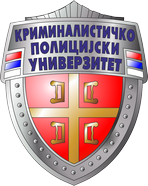UNIVERSITY OF CRIMINAL INVESTIGATION AND POLICE STUDIES

INFORMATION TECHNOLOGY

Course:

# Cryptology

Course Code: 1.29

ECTS: 6

Course Status:

Compulsory

Number of Effective Classes (Per Week): 3 + 2

Theoretical Education:

Practical Training:

Special Forms of Training:

3

2

-

Prerequisite/s: None

Educational Objective: Introduction to the concept and subject of cryptology. The subject provides the basics related to modular arithmetic and number theory, as well as an overview of algorithms for factorization of large numbers. Introduction to the basic characteristics of the method for cryptography and cryptanalysis.

Projected Outcome: The student acquires the necessary mathematical knowledge used in cryptography. Getting knowledge of a number of cryptographic algorithms and their application in cryptographic software for solving security problems.

Subject Contents

Theoretical education: An overview of the basis of number theory. Factoring algorithms. Modular arithmetic. Eliptic curves. Basic concepts and techniques of cryptological protection. Organization of the cryptological system. Classic cryptography. Data encryption algorithms. Symmetric and asymmetric algorithms. Generators of the pseudorandom sequences. Modern chain codes (stream chipers). Block codes: AES and DES, method of use. Private and public keys. Control sum. Hush function. Hash and MAC algorithms. RSA and ElGamal algorithms. Examples of cryptanalysis.

Practical training: Exercises follow theoretical lessons. Realizing examples of studied techniques in various available cryptologic programs.

Teaching Methods: modified lecture.

Assessment (Maximum Number of Points: 100)

Pre-Exam Obligations

No. of Points

Final Examination

No. of Points

Active participation in classes

10

Examination with practical problems and theoretical issues

50

Practical training

40

Textbook/s

1. Schneier, B. Primenjena kriptografija; Beograd : Mikroknjiga, 2007.

2. Živković, M. Kriptografija: skripta, http://poincare.matf.bg.ac.rs/~ezivkovm/nastava/kripto.pdf, 2010.

3. Salomaa, A. Public-key cryptography; Berlin: Springer, 1996.

4. Bauer, F. L. Decrypted secrets: methods and maxims of cryptology; Berlin: Springer, 1997.